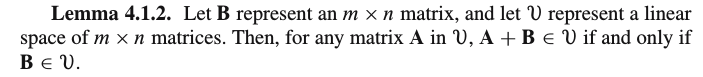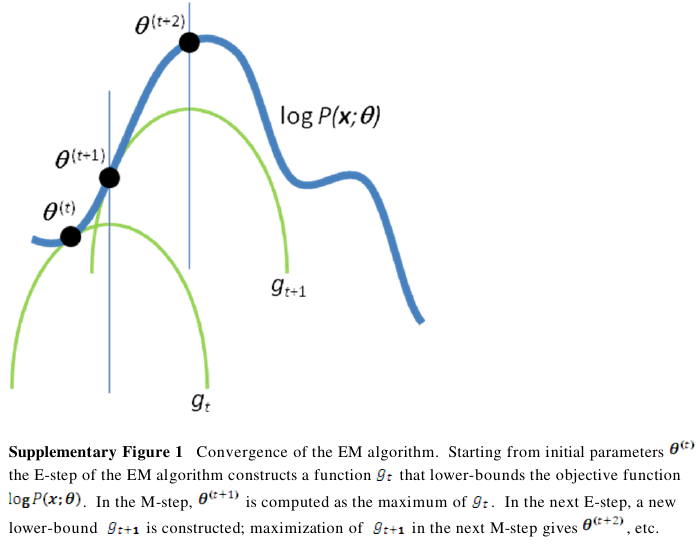## Inclusion and exclusion in linear spaces

Inclusion and exclusion in linear spacesThis can actually be generalized to the $aA + bB$ case. I will not provide a proof here, as it is quite straight-forward, but I would like to state an easy and interesting corollary:

Thm1. If $A \in U$ and $B \not\in U$, then $aA + bB \not\in U$.

This can be proved by contradiction.

With this under belt, we can prove the following theorem:

Thm2. Every linear space of n-vectors N has a basis.

Proof outline:

• $E_n = (e_1, e_2, \cdots, e_n)$ spans $N$
• The $N = R^n$ case is trivial, henceforth we assume $N \subset R^n$
• Then not all of $E_n$ is in $N$, otherwise we would have $N = R^n$
• Let's divide $E_n$ into two disjoint parts: $E_k = (e_1, \cdots, e_k)$ containing all $e_i$ in $N$ and $E_k' = (e_{k+1}, \cdots, e_n)$ containing the rest.
• $N \subset R^n = (s | a_i \in R) = (a_1e_1 + \cdots + a_ke_k + a_{k+1}e_{k+1} + \cdots + a_ne_n)$
• Let $T = (s | \text{at least one of } (a_{k+1} \cdots a_n) \text{ is not zero})$, then according Thm1 $N \cap T = \emptyset$, or $N-T = N$. Then if you subtract $T$ from both sides from the eq in the previous step, we have \begin{align*} N &\subset R^n - T \\ &= (s | a_i \in R) \\ &= (a_1e_1 + \cdots + a_ke_k + 0 + \cdots) \\ &= (a_1e_1 + \cdots + a_ke_k) \\ \end{align*} which is to say $(e_1, \cdots, e_k)$ spans $N$.
• But the set $(e_1, \cdots, e_k)$ is linearly indepent, hence we have found a basis for $N$

## Mathematical principles behind Expectation Maximization (EM) algorithm and a simple implementation in R

Mathematical principles behind Expectation Maximization (EM) algorithm and a simple implementation in R

### Disclaimer

This is an assignment report for the "Missing values in data analysis " course.

Mathematical formulations are roughly based on the paper by Chuong B Do and Serafim Batzoglou, "What is the expectation maximization algorith?", with some modification and clarification, which in my humble opinion lead to a better organized exposition. The R functions are independent work by me.

### The coin tossing example

Suppose we have two coins a and b, each with the probability of getting heads $\theta_a$ and $\theta_b$, but has the identical appearance, so we don't in advance which is which.

The experiment is designed like this: one coin is randomly drawn out and tossed nToss times, the number of heads is recorded. Suppose we repeat the experiment twice, and get a vector of the number of heads: $(x_1, x_2)$.

With the given data $(x_1, x_2)$, our goal is to estimate the probability of getting heads for each of these two experiments, i.e. the vector $(\theta_1, \theta_2)$, while having no information about which coin has been drawn. The EM algorithm will be helpful here.

### Mathematical formulations

Let $z$ be a vector of coin identity information. Obviously there are four possible configurations for $z$: \begin{align*} z = \begin{cases} (a, a) \\ (a, b) \\ (b, a) \\ (b, b) \\ \end{cases} \end{align*}

It follows that the likelihood function also has four cases: (To simplify the matters, let's take $x_1=2, \; x_2=9$ and let nToss for each experiment be 10.)

\begin{align*} P(x, z, \theta) = \begin{cases} \theta_a^2 (1-\theta_a)^8 \theta_a^9 (1-\theta_a) \\ \theta_a^2 (1-\theta_a)^8 \theta_b^9 (1-\theta_b) \\ \theta_b^2 (1-\theta_b)^8 \theta_a^9 (1-\theta_a) \\ \theta_b^2 (1-\theta_b)^8 \theta_b^9 (1-\theta_b) \\ \end{cases} \end{align*} And the conditional density for each of these cases is \begin{align*} P(z_i | x; \; \theta) = \frac{ P(x, z_i; \; \theta) }{ \sum_z P(x, z_i; \; \theta) } \end{align*}

The log-likelihood function is

\begin{align*} l(\theta) = \log \sum_z P(x, z_i; \theta) \end{align*}

Now we need a trick called Jensen's inequality here. This inequality states that for any concave function $h$, $h(E(y)) \ge E(h(y))$, since the log function is concave, this translates into $log(E(y)) \ge E(log(y))$ in our case.

Here comes the tricky part, we will manipulate the form of $l(\theta)$ a little. Suppose $D$ is any density of $z$, then

\begin{align*} l(\theta) &= \log \sum_z D \frac{P(x, z_i; \theta)}{D} \\ &= \log E(\frac{P(x, z; \theta)}{D}) \end{align*}

You see this is the perfect timing for Jensen's inequality:

\begin{align*} l(\theta) &= \log E(\frac{P(x, z; \theta)}{D}) \\ &\ge E(\log(\frac{P(x, z; \theta)}{D})) \\ &= \sum_z D \log(\frac{P(x, z; \theta)}{D}) \\ \end{align*}

Remember we have a convinient choice for $D$: the conditional density of $z_i$: $P(z_i | x; \; \theta)$, and substituting D with this, we get:

\begin{align*} l(\theta) &\ge \sum_z P(z_i | x; \; \theta) \log(\frac{P(x, z; \theta)}{P(z_i | x; \; \theta)}) \\ &= g(\theta) \\ \end{align*}

And $g$ is the estimation function that always lower bounds the likelihood function (i.e. always less than or equal to it). This is crucial in the EM algorithm, it starts with an arbitrary value of $\theta$, find a new theta that maximizes the lower-bounding function $g$, then update $g$ with the new theta, and repeat the two-step procedure until convergence. This can be best illustrated with a picture (copied from the aforementioned paper):In mathematical terms, this picture can be translated as:

\begin{align*} g(\hat{\theta}_0) &= \sum_z P(z_i | x; \; \hat{\theta}_0) \log(\frac{P(x, z; \theta)}{P(z_i | x; \; \hat{\theta}_0)}) \\ \hat{\theta}_1 &= \text{argmax}_{\theta} g(\hat{\theta}_0) \\ g(\hat{\theta}_1) &= \sum_z P(z_i | x; \; \hat{\theta}_1) \log(\frac{P(x, z; \theta)}{P(z_i | x; \; \hat{\theta}_1)}) \\ \hat{\theta}_2 &= \text{argmax}_{\theta} g(\hat{\theta}_1) \\ \vdots \\ \end{align*}

### A simple implementation in R

To examplify, we continue with our toy example. We simulate these two experiments in R and try to estimate $\theta$ with EM:

Double click to toggle code
#### test the optim function
## testopti = function(theta) {
##     (theta^2 + theta^2)
## }
## optim(c(.5, .2), method="L-BFGS-B", fn=testopti, lower=c(-1000, -1000), upper = c(1000, 1000))

nToss = 50
thetaTrue1 = .2
thetaTrue2 = .9
thetaTrue = c(thetaTrue1, thetaTrue2)
x1 = rbinom(1, nToss, thetaTrue1)
x2 = rbinom(1, nToss, thetaTrue2)
print(x1)
print(x2)
x = c(x1, x2)

# four possible configurations,
# coin1 coin1; coin1, coin2; ...
z1 = c(1, 1)
z2 = c(1, 2)
z3 = c(2, 1)
z4 = c(2, 2)
z = list(z1, z2, z3, z4)
print(z)

# likelihood function
lhfun = function(x, zi, theta) {
x1 = x
x2 = x
theta1 = theta[zi]
theta2 = theta[zi]
theta1^x1 * (1-theta1)^(nToss-x1) * theta2^x2 * (1-theta2)^(nToss-x2)
}

lhsum = function(x, z, theta) {
lhlist = lapply(z, function(zi) {
lhfun(x, zi, theta)
})
do.call(sum, lhlist)
}

pzi = function(x, z, zi, thetaHat) {
likelihoodSum = lhsum(x, z, thetaHat)
likelihoodZi = lhfun(x, zi, thetaHat)
likelihoodZi / likelihoodSum
}
sapply(1:length(z), function(i) {
pzi(x, z, z[[i]], c(.2, .8))
})

pzi(x, z, z[], c(.3, .8))

## estimation function
eFunc = function(x, z, thetaHat) {
toMaximize = function(theta) {
glist = lapply(1:length(z), function(i) {
pzires = pzi(x, z, z[[i]], thetaHat)
pzires * log(lhfun(x, z[[i]], theta) / pzires)
})
-do.call(sum, glist)
}
}
eFuncToMax = eFunc(x, z, c(.3, .7))
eFuncToMax(c(.3, .8))

optim(c(.2, .3), method="L-BFGS-B", fn=eFuncToMax, lower=c(0.001, 0.001), upper = c(.999, .999))

emSimple = function(x, z, thetaHatInit) {
thetaHat0 = optim(
# initial guess for the optimization procedure, not so important.
c(.2, .3),
method="L-BFGS-B",
fn=eFunc(x, z, thetaHatInit),
lower=c(0.001, 0.001),
upper = c(.999, .999)
)$par thetaHat1 = optim( c(.2, .3), method="L-BFGS-B", fn=eFunc(x, z, thetaHat0), lower=c(0.001, 0.001), upper = c(.999, .999) )$par
nIters = 1
while(max(abs(thetaHat1 - thetaHat0)) > 0.00001) {
nIters = nIters + 1
thetaHat0 = thetaHat1
thetaHat1 = optim(
c(.2, .3),
method="L-BFGS-B",
fn=eFunc(x, z, thetaHat0),
lower=c(0.001, 0.001),
upper = c(.999, .999)
)$par } list(EMestimate=thetaHat1, nIters) } emSimple(x, z, c(.5, .6))  I have set$nToss$to 50, the true theta to$(0.2, 0.9)$, and using the EM algorithm, my simple EM function estimates theta to be$(0.22, 0.94)\$ in just two iterations, which is amazingly close to the true theta:

Double click to toggle code
> emSimple(x, z, c(.5, .6))

 0.2200023 0.9399953

[]
 2